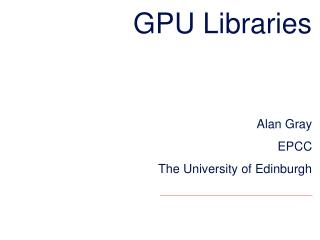# GPU Libraries - PowerPoint PPT PresentationDownload PresentationGPU Libraries

GPU LibrariesDownload Presentation## GPU Libraries

- - - - - - - - - - - - - - - - - - - - - - - - - - - E N D - - - - - - - - - - - - - - - - - - - - - - - - - - -
##### Presentation Transcript

1. GPU Libraries Alan Gray EPCC The University of Edinburgh

2. Overview • Motivation for Libraries • Simple example: Matrix Multiplication • Overview of available GPU libraries

3. Computational Libraries • There are many “common” computational operations that are relevant for multiple problems • It is not productive for each user to implement their own version from scratch • It is also usually very complex to implement in a way that gets optimal performance • Solution: re-usable libraries • User just integrates call to library function within code • Library implementation is optimised for platform in use • Obviously only works if desired library exists • Many CPU libraries have developed and in use for many years • An increasing number of GPU libraries are now available

4. Simple Example: Matrix Multiplication

5. Simple Example: Matrix Multiplication matrix1 matrix2 matrix3 for (i = 0; i < 2; i++) { for (j = 0; j < 2; j++) { matrix3[i][j]=0.; for (k = 0; k < 2; k++) { matrix3[i][j]+=matrix1[i][k]*matrix2[k][j]; } } }

6. Matrix multiplication for large N • Each element of the result matrix is built up as the sum of a number of multiplications • This naïve implementation is not the only order in which the sum can be accumulated • It is much faster (when N is large) to rearrange the nested loop structure such that small sub blocks of matrix1 and matrix2 are operated on in turn • Because these can be kept resident in fast on-chip caches and/or registers • Removes memory access bottlenecks for (i = 0; i < N; i++) { for (j = 0; j < N; j++) { matrix3[i][j]=0.; for (k = 0; k < N; k++) { matrix3[i][j]+=matrix1[i][k]*matrix2[k][j]; } } }

7. Linear Algebra Libraries • Matrix multiplication (and similar) can be implemented easily by hand, but results will be sub-optimal • The Basic Linear Algebra Subprograms (BLAS) has been around since 1979, and provides a range of basic linear algebra operations • With implementations optimised for modern CPUs • cuBLAS, a GPU-accelerated implementation, is available as part of the CUDA distribution • Other more complex linear algebra operations, e.g. matrix inversion, eigenvalue determination… (built out of multiple BLAS operations), and are available in LAPACK (CPU) • with MAGMA (free) and CULA (commercial) being two alternative GPU-accelerated implementations

8. cuBLAS Matrix Multiplication • First, note that cuBLASuses linear indexing with column-major storage • 2D arrays need to be “flattened” intld = N // leading dimension for (i = 0; i < N; i++) { for (j = 0; j < N; j++) { matrix3[i*ld+j]=0.; for (k = 0; k < N; k++) { matrix3[i*ld+j]+=matrix1[i*ld+k]*matrix2[k*ld+j]; } } }

9. cuBLAS Matrix Multiplication http://docs.nvidia.com/cuda/cublas

10. cuBLAS Matrix Multiplication • For our simple 2x2 example earlier double alpha=1.0; double beta=0.0; intld=2; //leading dimension int N=2; cublasHandle_thandle; cublasCreate(&handle); //allocate memory for d_matrix1, d_matrix2, and d_matrix3 on GPU // copy data to d_matrixand d_matrix2 on GPU cublasDgemm(handle,CUBLAS_OP_N,CUBLAS_OP_N, N, N, N, &alpha, d_matrix1, ld, d_matrix2, ld, &beta, d_matrix3, ld); //also some additional code needed to ensure success of operation //copy result d_matrix3 back from GPU //free GPU memory //cublasDestroy(handle);

11. GPU Accelerated Libraries • developer.nvidia.com/gpu-accelerated-libraries